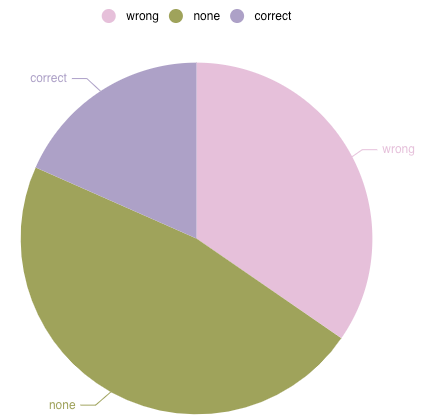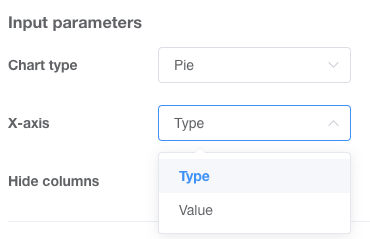# Introduction

The Pie Chart displays data of one series in a pie with each piece being the size of proportioned amount of the total amount:# Expected Data and Graph Designer

The Pie Chart expects data in a two column format such as:

TypeValue
wrong64
none87
correct34

In the Graph Designer you can then select the column you want to use for your x-axis, which in this case is the different sections of the pie:Hence, the x-axis column does not need to be the first column. However, for consistency purposes it is recommended to always use your first column for the x-axis.

The size of each pie piece will be automatically calculated and if you activate the tooltip, which you can do in the Graph Designer:You can see the exact percentage as well as the absolute amount for each pie piece: